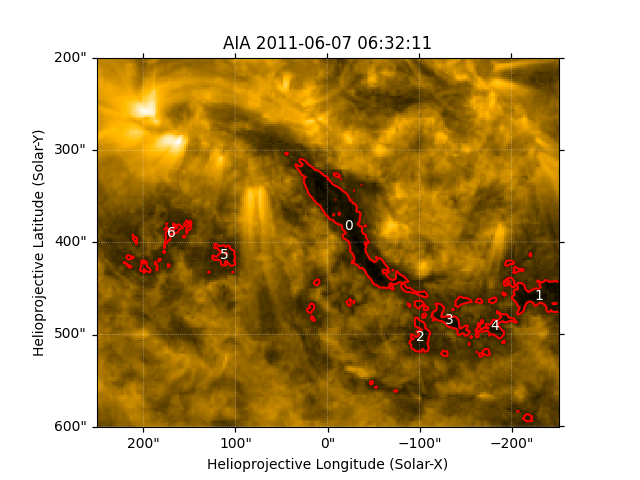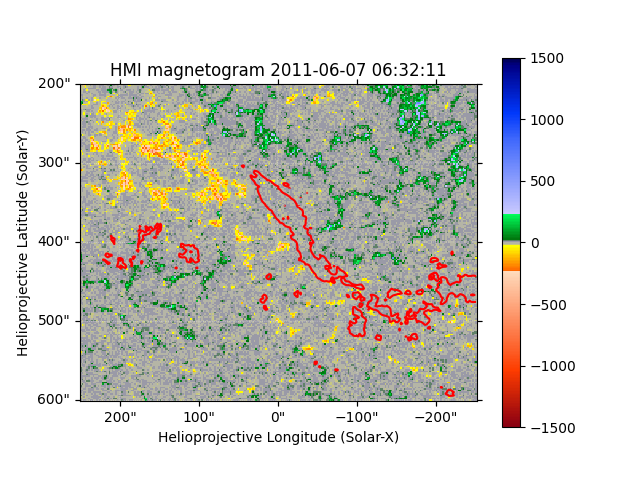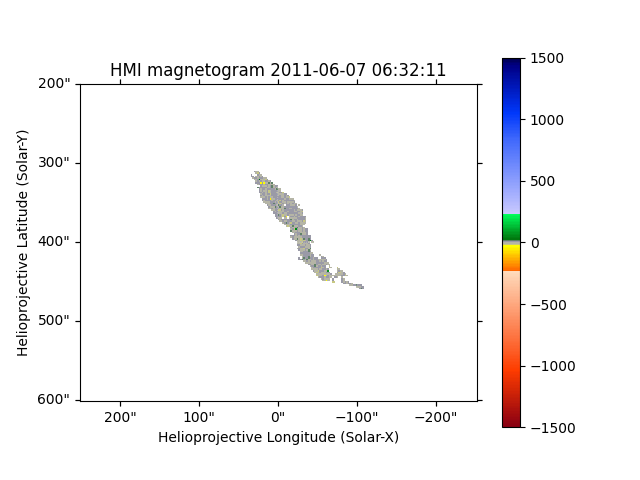# Masking HMI based on the intensity of AIA#

In this example we will demonstrate how to mask out regions within a HMI image based on the intensity values of AIA.

import matplotlib.pyplot as plt
import numpy as np
from matplotlib.colors import Normalize
from skimage.measure import label, regionprops

import astropy.units as u
from astropy.coordinates import SkyCoord

import sunpy.map
from sunpy.data.sample import AIA_171_IMAGE, HMI_LOS_IMAGE


We will use an AIA 171 image from the sample data and crop it to capture a region of interest.

aia = sunpy.map.Map(AIA_171_IMAGE)
aia = aia.submap(
bottom_left=SkyCoord(-250, 200, unit=u.arcsec, frame=aia.coordinate_frame),
width=500 * u.arcsec,
height=400 * u.arcsec,
)


Next we call the reproject_to() to reproject the HMI Map to have exactly the same grid as the AIA Map. Aligning AIA and HMI Data with Reproject provides more reference.

hmi = sunpy.map.Map(HMI_LOS_IMAGE)
hmi = hmi.reproject_to(aia.wcs)
hmi.nickname = 'HMI magnetogram'


Now we will identify separate regions below a threshold in the AIA Map. In this case, we want the darker patches that have pixel values below 200. Then, using skimage, we can label() and calculate the properties of each region using regionprops().

segmented = aia.data < 200
labeled = label(segmented)
regions = regionprops(labeled, hmi.data)
# We want the largest region, so we will sort by descending order in size.
regions = sorted(regions, key=lambda r: r.area, reverse=True)


Now to plot and label the first 7 regions seen in AIA with the region “0” being the largest.

fig = plt.figure()
aia.plot(axes=ax)
aia.draw_contours(axes=ax, levels=200 * u.ct, colors="r")
for i in range(7):
plt.text(*np.flip(regions[i].centroid), str(i), color="w", ha="center", va="center")Now let’s plot those same regions on the reprojected HMI Map.

fig = plt.figure()
im = hmi.plot(axes=ax, cmap="hmimag", norm=Normalize(-1500, 1500))
aia.draw_contours(axes=ax, levels=200 * u.ct, colors="r")
fig.colorbar(im)<matplotlib.colorbar.Colorbar object at 0x7f2e0bc75120>


Now we have the regions, we need to create a new HMI map that masks out everything but the largest region. To do so, we need to create the mask from the bounding box returned by skimage.

bbox = regions.bbox
mask[bbox: bbox, bbox: bbox] = ~regions.image


Finally, plot the largest HMI region.

fig = plt.figure()